# GLSL Shader Examples: Blue Color Screen

Create a shader which fills the screen with blue color. For the space occupied by right half of screen, introduce a vertical green bar located in the middle of it, with its width being equal to one-third of it. This should result in right half of screen being divided into three equal parts (blue-green-blue). As for the left half of screen, introduce a pair of vertical red bars, with width of each being equal to one-fourth of it, resulting in left half of screen being divided into four equal parts (blue-red-blue-red).

SOLUTION:

In this sample we are going to create a basic shader in GLSL language. GLSL (OpenGL Shading Language, also referred to as GLslang), is a shading language with C-like syntax, used for programming shader effects. For the sake of demonstration purposes we will use shadertoy.com (the shader code itself is going to be tested at shadertoy.com/new )

First of all, we need to fill the screen with blue color. Shade returns four numerical values, corresponding to colors red/green/blue, as well as alpha (transparency level). So by setting each of colors to 0 we can get a black screen:

```void mainImage( out vec4 fragColor, in vec2 fragCoord )
{
fragColor = vec4(0,0,0,1);
}
```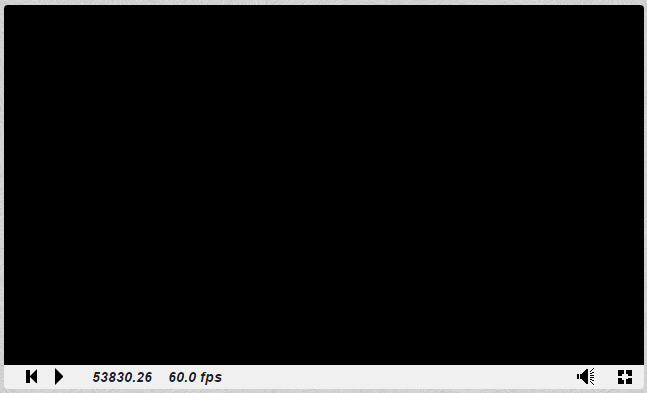Now we need to raise numberical value of blue color from lowest (0) to 1. Note that inputting values in-between of thes extremes (for example 0.32) is still possible.

```void mainImage( out vec4 fragColor, in vec2 fragCoord )
{
fragColor = vec4(0,0,1,1);
}
```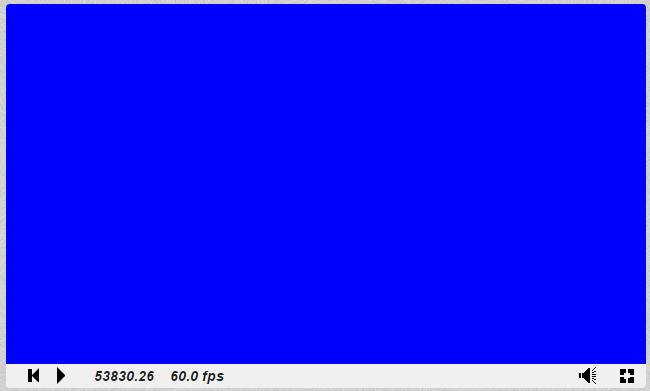We got the blue screen, and now we need to determine its middle point. First of all lets create new variable of vec2 type (for storing the coordinates), and another one of vec4 type (for storing the color information)

```void mainImage( out vec4 fragColor, in vec2 fragCoord )
{
//this is the default blue color
vec4 varcolor = vec4(0,0,1,1);
//pixel coordinates obtained
vec2 varcoord = fragCoord.xy;
/* x coordinate divided across screen size,
so that we could refer to coordinates as a set of values from 0.0 to 1.0 */
varcoord.x = varcoord.x / iResolution.x;
//now we can make a rule where starting from exactly the middle of screen
if(varcoord.x > 0.5) {
//light blue color is used instead (we increased green color value by 0.5 for this)
varcolor = vec4(0,0.5,1,1);
}
//displaying the result on screen now
fragColor = varcolor;
}
```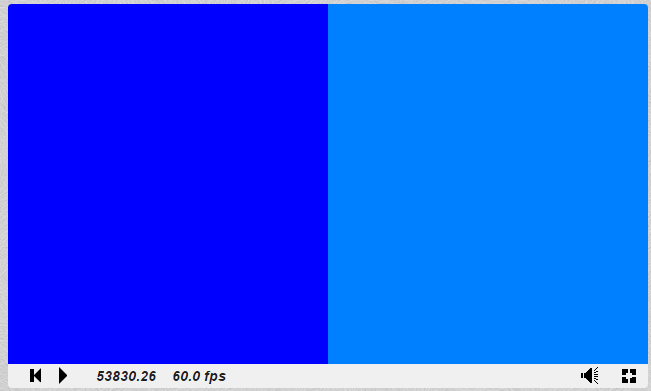Now we have determined that the varcoord.x values from 0.5 to 1.0 represent the right half of the screen. We can use this knowledge in order to install the vertical green bar in the middle third of this particular screen half:

```void mainImage( out vec4 fragColor, in vec2 fragCoord )
{
vec4 varcolor = vec4(0,0,1,1);
vec2 varcoord = fragCoord.xy;
varcoord.x = varcoord.x / iResolution.x;
//this variable holds the length of one third of screen's half
float thirdOfAHalf = 0.5/3.0;
if(varcoord.x > 0.5) {
varcolor = vec4(0,0.5,1,1);
}
if(varcoord.x > (0.5 + thirdOfAHalf) && varcoord.x < (1.0 - thirdOfAHalf))
{
varcolor = vec4(0,1,0,1);
}
fragColor = varcolor;
}
```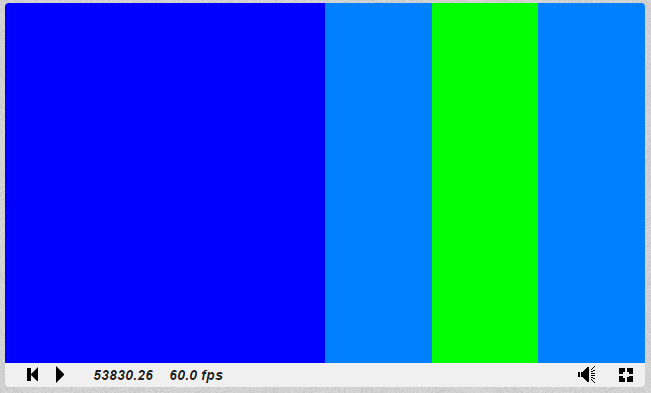Similarly, we can add a pair of red bars to the left half of the screen:

```void mainImage( out vec4 fragColor, in vec2 fragCoord )
{
vec4 varcolor = vec4(0,0,1,1);
vec2 varcoord = fragCoord.xy;
varcoord.x = varcoord.x / iResolution.x;
//this variable holds the length of one third of screen's half
float thirdOfAHalf = 0.5/3.0;
//this variable holds the length of one fourth of screen's half
float fourthOfAHalf = 0.5/4.0;
if(varcoord.x > 0.5) {
varcolor = vec4(0,0.5,1,1);
}
if(varcoord.x > (0.5 + thirdOfAHalf) && varcoord.x < (1.0 - thirdOfAHalf)) { varcolor = vec4(0,1,0,1); } //adding first red bar f(varcoord.x > fourthOfAHalf && varcoord.x < 2.0*fourthOfAHalf) { varcolor = vec4(1,0,0,1); } //adding second red bar. We could just write “0.5” instead of “4.0*fourthOfAHalf” if(varcoord.x > 3.0*fourthOfAHalf && varcoord.x < 4.0*fourthOfAHalf)
{
varcolor = vec4(1,0,0,1);
}
fragColor = varcolor;
}
```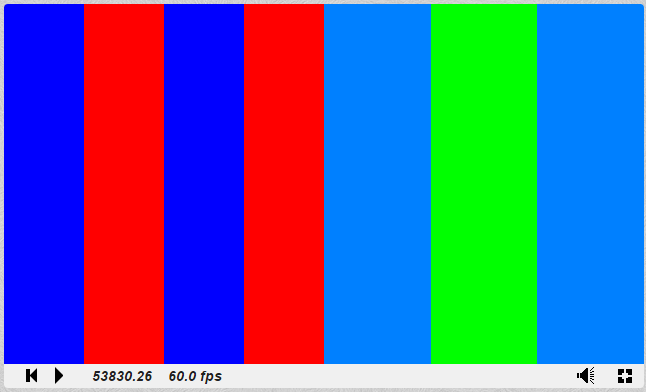Now when the additional color bars are in place, we no longer need the light blue color to tell apart different halves of screen, so we can remove it and streamline our code in the following fashion:

```void mainImage( out vec4 fragColor, in vec2 fragCoord )
{
vec4 varcolor = vec4(0,0,1,1);
vec2 varcoord = fragCoord.xy;
varcoord.x = varcoord.x / iResolution.x;
float thirdOfAHalf = 0.5/3.0;
float fourthOfAHalf = 0.5/4.0;
if(varcoord.x > (0.5 + thirdOfAHalf) && varcoord.x < (1.0 - thirdOfAHalf)) varcolor = vec4(0,1,0,1); if(varcoord.x > fourthOfAHalf && varcoord.x < 2.0*fourthOfAHalf) varcolor = vec4(1,0,0,1); if(varcoord.x > 3.0*fourthOfAHalf && varcoord.x < 0.5)
varcolor = vec4(1,0,0,1);
fragColor = varcolor;
}
```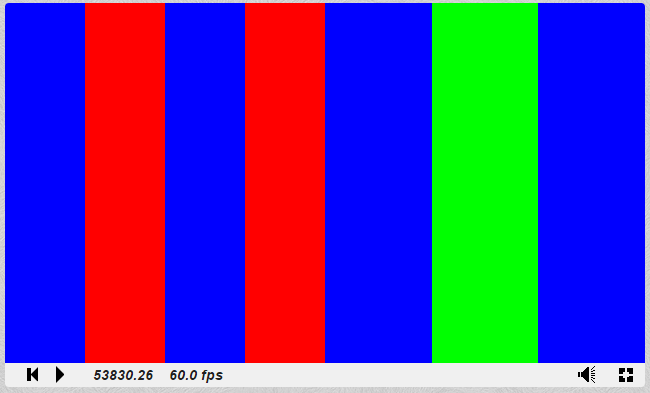We hope you like our GLSL shader examples. If it is so we would like to offer you our service. AssignmentShark is a service that helps students to reach their academic goals. It was established especially for students which need to complete technical tasks. On our website you have the ability to choose the most appropriate expert and cooperate with him or her. We hire only the best experts from different spheres so that you can be confident that you will submit correct assignments. Look through other our sample assignments and you’ll make sure that all of our experts are good in their field. Or, you can just make the order right now and make your final opinion through your own experience!